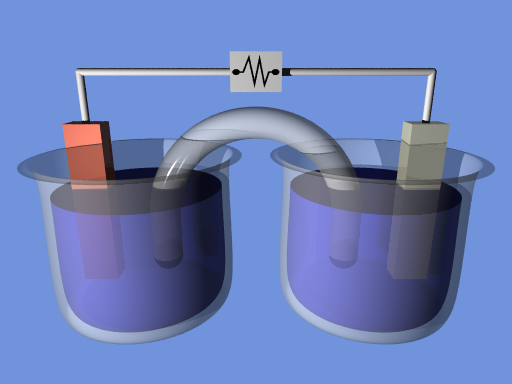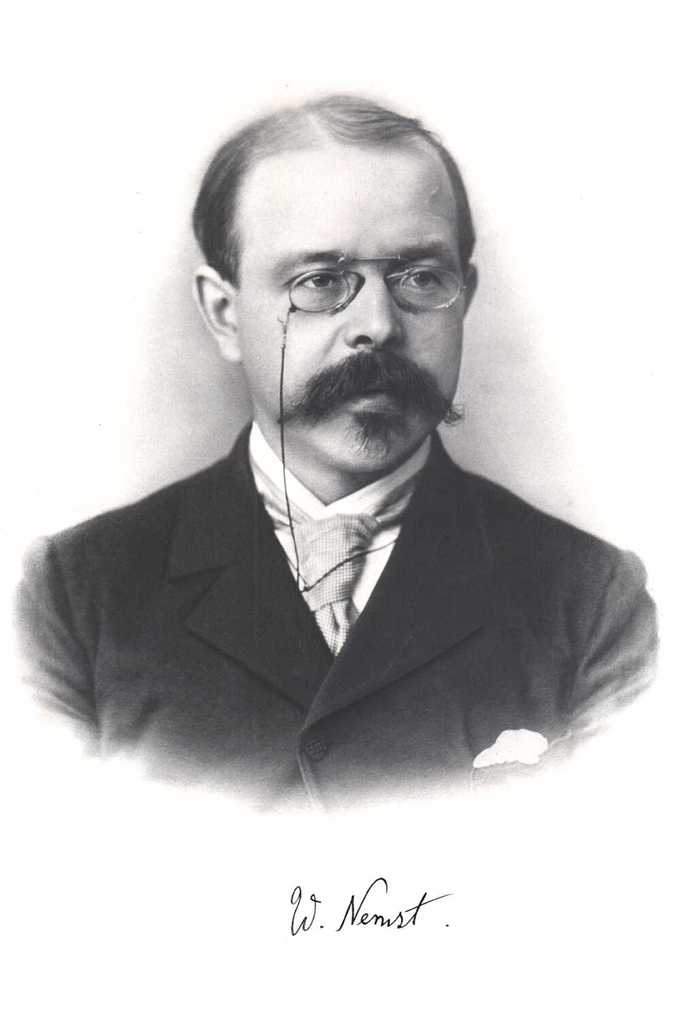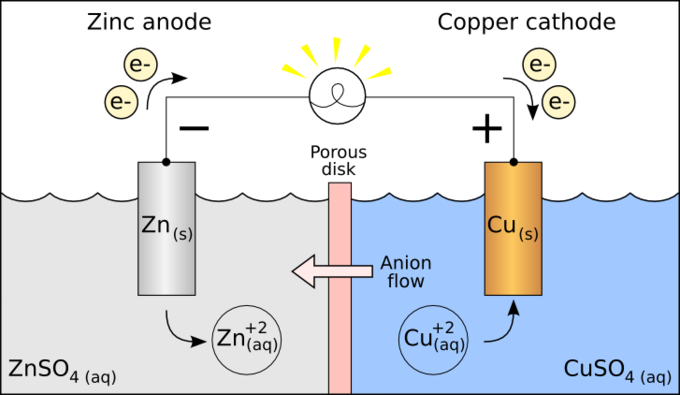## The Nernst Equation

In electrochemistry, the Nernst equation can be used to determine the reduction potential of an electrochemical cell.

### Learning Objectives

Recall the Nernst equation

### Key Takeaways

#### Key Points

• In electrochemistry, the Nernst equation can be used to determine the reduction potential of a half-cell in an electrochemical cell.
• The Nernst equation can also be used to determine the total voltage ( electromotive force ) for a full electrochemical cell.
• The Nernst equation gives a formula that relates the numerical values of the concentration gradient to the electric gradient that balances it.

#### Key Terms

• electrochemistry: The science of the chemistry associated with the flow of electricity, especially at the surface of an electrode.
• electrochemical cell: A container containing an electrolyte and two electrodes; used to produce direct current electricity. One or more of them constitute a battery.
• voltage: The amount of electrostatic potential between two points in space.
• electromotive force: Voltage generated by a battery or by a varying magnetic field.

In electrochemistry, the Nernst equation can be used, in conjunction with other information, to determine the reduction potential of a half-cell in an electrochemical cell. It can also be used to determine the total voltage, or electromotive force, for a full electrochemical cell. It is named after the German physical chemist who first formulated it, Walther Nernst.Electrochemical cell: Schematic of an electrochemical cell.

The Nernst equation gives a formula that relates the electromotive force of a nonstandard cell to the concentrations of species in solution:

$\text{E} = \text{E}^\text{o} - \frac {0.0257}{\text{n}} \text{ln}\ \text{Q}$

In this equation:

• E is the electromotive force of the non-standard cell
• Eo is the electromotive force of the standard cell
• n is the number of moles of electrons transferred in the reaction

ln Q is the natural log of $\frac{\text{C}^\text{c}\text{D}^\text{d}}{\text{A}^\text{a}\text{B}^\text{b}}$, where the uppercase letters are concentrations, and the lowercase letters are stoichiometric coefficients for the reaction: $\text{aA} + \text{bB} \rightarrow \text{cC} + \text{dD}$

### Example

Find the cell potential of a galvanic cell based on the following reduction half-reactions where [Ni2+] = 0.030 M and [Pb2+] = 0.300 M.

Ni2+ + 2 e- → Ni, E0 = -0.25 V

Pb2+ + 2 e- → Pb, E0 = -0.13 V

First, find the electromotive force for the standard cell, which assumes concentrations of 1 M.

In order for this reaction to run spontaneously (positive Eo cell) the nickel must be oxidized and therefore its reaction needs to be reversed. The added half-reactions with the adjusted E0 cell are:

$\text{Pb}^{2+} + \text{Ni} \rightarrow \text{Ni}^{2+} + \text{Pb}, \ \ \ \text{E}^\text{o} = 0.12\ \text{V}$

The number of moles of electrons transferred is 2 and Q is $\frac{[\text{Ni}^{2+}][\text{Pb}]}{[\text{Pb}^{2+}][\text{Ni}]}$, where Pb and Ni are pure solids whose concentrations remain constant, so they are dropped from the equation.

$\text{E} = \text{E}^\text{o} - \frac {0.0257}{\text{n}} \text{ln}\ Q$

$\text{E} = 0.12\text{V} - \frac {0.0257}{2} \text{ln}\frac{0.030}{0.300}$

$\text{E} = 0.15\ \text{V}$

## Concentration of Cells

Walther Nernst proposed a mathematical model to determine the effect of reactant concentration on the electrochemical cell potential.

### Learning Objectives

Discuss the implications of the Nernst equation on the electrochemical potential of a cell

### Key Takeaways

#### Key Points

• In the 20th century, German chemist Walther Nernst proposed a mathematical model to determine the effect of reactant concentration on electrochemical cell potential.
• In the late 19th century, Josiah Willard Gibbs formulated a theory to predict whether a chemical reaction would be spontaneous based on free energy: $\Delta \text{G} = \Delta \text{G}^\text{o} + \text{RT} \times \text{ln}\ \text{Q}$.
• Based on Gibbs’ work, Nernst extended the theory to include the contribution from electric potential on charged species. The change in Gibbs free energy for an electrochemical cell can be related to the cell potential. Gibbs’ theory is: $\Delta \text{E} = \Delta \text{E}^\text{o} - \frac{\text{RT}}{\text{nF}} \times \text{ln}\ \text{Q}$.

#### Key Terms

• electrochemical cell: A container containing an electrolyte and two electrodes; used to produce direct current electricity. One or more of them constitute a battery.
• free energy: The difference between the internal energy of a system and the product of its entropy and absolute temperature.

### Gibbs Free Energy and Cell Potential

The standard potential of an electrochemical cell requires standard conditions for all of the reactants. When reactant concentrations differ from standard conditions, the cell potential will deviate from the standard potential. In the late 19th century, Josiah Willard Gibbs formulated a theory to predict whether a chemical reaction would be spontaneous based on free energy:

$\Delta \text{G} = \Delta \text{G}^\text{o} + \text{RT} \times \text{ln}\ \text{Q}$

Here, ΔG is the change in Gibbs free energy, T is absolute temperature, R is the gas constant, and Q is the reaction quotient. In chemistry, a reaction quotient is a function of the activities or concentrations of the chemical species involved in a chemical reaction. Gibbs’ key contribution was to formalize the understanding of the effect of reactant concentration on spontaneity.

In the 20th century, German chemist Walther Nernst extended Gibbs’ theory to include the contribution from electric potential on charged species. The change in Gibbs free energy for an electrochemical cell can be related to the cell potential. Therefore, Gibbs’ theory is:

$\text{nF}\Delta \text{E} = \text{nF}\Delta \text{E}^\text{o} - \text{RT}\times \text{ln}\ \text{Q}$

Here, n is the number of moles of electrons, F is the Faraday constant ($\frac {\text{Coulombs}}{\text{mole}}$), and ΔE is the cell potential.Walther Nernst: A portrait of Walther Nernst

### The Nernst Equation

Finally, Nernst divided by the amount of charge transferred to arrive at a new equation that now bears his name. The Nernst equation is:

$\Delta \text{E} = \Delta \text{E}^\text{o} - \frac{\text{RT}}{\text{nF}} \times \text{ln}\ \text{Q}$

The Nernst equation can be used to calculate the output voltage changes in a pair of half-cells under non-standard conditions.A typical galvanic electrochemical cell: Under standard conditions, the output of this pair of half-cells is well known. When a change in the concentration or activity of reactants occurs, or the temperature or pressure changes, the output voltage changes. It is calculated via the Nernst equation.

## Free Energy and Cell Potential

In a galvanic cell, where a spontaneous redox reaction drives the cell to produce an electric potential, the change in Gibbs free energy must be negative.

### Learning Objectives

Calculate the change in Gibbs free energy of an electrochemical cell, and discuss its implications for whether a redox reaction will be spontaneous

### Key Takeaways

#### Key Points

• In a galvanic cell, the Gibbs free energy is related to the potential by: ΔG°cell = −nFE°cell.
• If E°cell > 0, then the process is spontaneous (galvanic cell).
• If E°cell < 0, then the process is nonspontaneous ( electrolytic cell).

#### Key Terms

• galvanic cell: Electrochemical cell that derives electrical energy from spontaneous redox reaction taking place within the cell.
• electrode potential: The potential difference of a half-reaction that occurs across a reversible cell made up of any electrode and a standard hydrogen electrode.
• Gibbs free energy: The difference between the enthalpy of a system and the product of its entropy and absolute temperature; a measure of the useful work obtainable from a thermodynamic system at constant temperature and pressure.
• oxidizing agent: Any substance that oxidizes, or receives electrons from, another; in so doing, it becomes reduced.

The basis for an electrochemical cell, such as the galvanic cell, is always a redox reaction that can be broken down into two half-reactions: oxidation occurs at the anode, where there is a loss of electrons, and reduction occurs at the cathode, where there is a gain of electrons. Electricity is generated due to the electric potential difference between two electrodes. This is related to how easily the oxidized species gives up electrons and how badly the reduced species wants to gain them.Electrochemical cell: A demonstration electrochemical cell setup resembling the Daniell cell. The two half-cells are linked by a salt bridge carrying ions between them. Electrons flow in the external circuit.

In electrochemistry, the standard electrode potential, abbreviated E°, is the measure of the individual potential of a reversible electrode at standard state, which is with solutes at an effective concentration of 1 M, and gases at a pressure of 1 atm. The values are most often tabulated at 25 °C (298 K).

### Is It Oxidized or Reduced?

Since the standard electrode potentials are given in their ability to be reduced, the bigger the standard reduction potentials, the easier they are to be reduced; in other words, they are simply better oxidizing agents. For example, F2 has a potential of 2.87 V and Li+ has a potential of -3.05 V. F2 reduces easily and is therefore a good oxidizing agent. In contrast, Li(s) would rather undergo oxidation, so it is a good reducing agent.

In the example of Zn2+, whose standard reduction potential is -0.76 V, it can be oxidized by any other electrode whose standard reduction potential is greater than -0.76 V and can be reduced by any electrode with standard reduction potential less than -0.76 V.

### The Sign of the change in Gibbs Free Energy

In a galvanic cell, where a spontaneous redox reaction drives the cell to produce an electric potential, the change in Gibbs free energy must be negative. This is the opposite of the cell potential, which is positive when electrons flow spontaneously through the electrochemical cell. The relationship between $\Delta \text{G}^\text{o}$ and $\text{E}^\text{o}$ is given by the following equation:

$\Delta \text{G}^\text{o} = -\text{nFE}^\text{o}$

Here, n is the number of moles of electrons and F is the Faraday constant (96,485$\frac {\text{Coulombs}}{\text{mole}}$). As such, the following rules apply:

• If E°cell > 0, then the process is spontaneous (galvanic cell)
• If E°cell < 0, then the process is non-spontaneous (the voltage must be supplied, as in an electrolytic cell)

### Example

Calculate the change in Gibbs free energy of an electrochemical cell where the following redox reaction is taking place:

$\text{Pb}^{2+} + \text{Ni} \rightarrow \text{Ni}^{2+} + \text{Pb} \ \ \ \ \ \ \ \ \text{E}^\text{o} = 0.12\ \text{V}$

To solve, we need to use the equation:

$\Delta \text{G}^\text{o} = -\text{nFE}^\text{o}$

The number of moles of electrons transferred is 2, while the cell potential is equal to 0.12 V. One volt is $1\frac {\text{Joule}}{\text{Coulomb}}$.

$\Delta \text{G}^\text{o} = -2 \ \text{moles}\ \text{e}^- \times 96485\frac {\text{Coulombs}}{\text{mole}} \times 0.12 \frac {\text{Joules}}{\text{Coulomb}}$

$\Delta \text{G}^\text{o} = -23,156 \ \text{J}\ = -2.3 \ \text{kJ}$

Because the change in Gibbs free energy is negative, the redox process is spontaneous.

## Equilibrium Constant and Cell Potential

The equilibrium constant K can be calculated using the Nernst equation.

### Learning Objectives

Calculate the equilibrium constant, K, for a galvanic cell using the Nernst equation

### Key Takeaways

#### Key Points

• In an electrochemical cell, each chemical species will move from areas with higher electrochemical potential to areas with lower electrochemical potential.
• The Nernst equation can be used to determine the equilibrium reduction potential of a half-cell in an electrochemical cell.
• The Nernst equation can also be used to determine the total voltage, or electromotive force, for a full electrochemical cell.

#### Key Terms

• Nernst equation: Used to determine the equilibrium reduction potential of a half-cell in an electrochemical cell, as well as the total voltage for a full electrochemical cell.

### Electric and Chemical Potential

Each chemical species has an electrochemical potential (quantity with units of energy ) at any given location, which represents how easy or difficult it is to add more of that species to that location. If possible, a species will move from areas with higher electrochemical potential to areas with lower electrochemical potential. In equilibrium, the electrochemical potential will be constant everywhere for each species. There might be a different value for each different species.

For example, if a glass of water has sodium ions (Na+) dissolved uniformly in it, and an electric field is applied across the water, then the sodium ions will tend to get pulled by the electric field toward one side. We say the ions have electric potential energy, and are moving to lower their potential energy.

Likewise, if a glass of water has a lot of dissolved sugar on one side and none on the other side, each sugar molecule will randomly diffuse around the water until there is and equal concentration of sugar everywhere. We say that the sugar molecules have a ” chemical potential,” which is higher in the high-concentration areas, and the molecules move to lower their chemical potential.

These two examples show that an electrical potential and a chemical potential can both give the same result: a redistribution of the chemical species. Therefore, it makes sense to combine them into a single “potential,” the electrochemical potential, which can directly give the net redistribution by taking both into account.

### The Nernst Equation and Electrochemical Potential

In electrochemistry, the Nernst equation can be used, in conjunction with other information, to determine the equilibrium reduction potential of a half-cell. It can also be used to determine the total voltage, or electromotive force, for a full electrochemical cell. The Nernst equation gives a formula that relates the numerical values of the concentration gradient to the electrical gradient that balances it.

For example, let’s say a concentration gradient was established by dissolving KCl in half of a divided vessel that was originally full of H2O. A membrane permeable to K+ ions was introduced between the two halves. Empirically, an equilibrium situation would arise where the chemical concentration gradient could be balanced by an electrical gradient that opposes the movement of charge. In other words, a voltage could be applied to stop the movement of the K+ ions.

We can also consider an example where the solutions are CuSO4 and ZnSO4. Each solution has a corresponding metal strip in it, and a salt bridge or porous disk connecting the two solutions. This allows SO42- ions to flow freely between the copper and zinc solutions. In order to calculate the standard potential, we have to look up the half-reactions of copper and zinc.

The standard cell potential for the reaction is then +0.34 V – (-0.76 V) = +1.10 V. The polarity of the cell is determined by knowing that zinc metal is a stronger reducing agent than copper metal. Therefore, the standard reduction potential for zinc is more negative than that of copper. Thus, zinc metal will lose electrons to copper ions and develop a positive electrical charge.Galvanic cell: Schematic of a galvanic cell for the reaction between Zn and Cu.

The cell equilibrium constant, K, can be derived from the Nernst equation:

$\text{E}=\text{E}^\text{o}−\frac{\text{RT}}{\text{nF}}\text{ln}\text{Q}$

In this equation, E is the cell potential, Eo is the standard cell potential (i.e., measured under standard conditions), F is Faraday ‘s constant, R is the universal gas constant, T is the temperature in degrees Kelvin, Q is the reaction quotient (which has the same algebraic from as the equilibrium constant expression, except it applies to any time during the reaction’s progress), and n is the number of moles of electrons that are transferred in the balanced chemical equation of the redox process.

The cell potential is zero at equilibrium (E=0), and Q (the reaction quotient) can now be designated as the equilibrium constant K. Solving for lnK, we obtain:

$\text{ln}\ \text{K} = \frac {\text{nFE}^\text{o}}{\text{RT}}$

### Example

Calculate the equilibrium constant K, from the following reaction studied at a temperature of 298K:

$\text{Zn}^{2+} + \text{Cu} \rightarrow \text{Cu}^{2+} + \text{Zn} \ \ \ \ \ \ \ \ \ \ \ \ \ \ \ \text{E}^\text{o} = 1.10\ \text{V}$

$\text{ln}\ \text{K} = \frac {\text{nFE}^\text{o}}{\text{RT}}$

In this problem, 2 moles of electrons are being transferred, F is 96485 $\frac {\text{Coulombs}}{\text{mole}}$, R is 8.31$\frac {\text{Joules}}{\text{K} \times \text{mole}}$, T is 298 K, and Eo is 1.10$\frac {\text{Joules}}{\text{Coulomb}}$.

Plugging the values into the equation, we get:

$\text{ln}\ \text{K} = \frac {2 \times 96485 \times 1.10}{8.31\times 298}$

$\text{ln}\ \text{K} = 85.7$

Solving for K, we get:

K = e85.7

K = 1.68 x 1037

This is a particularly high equilibrium constant value, indicating that the equilibrium strongly favors the formation of products (the reaction is effectively irreversible towards the formation of products).